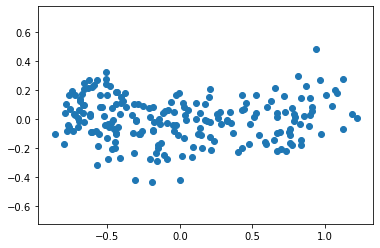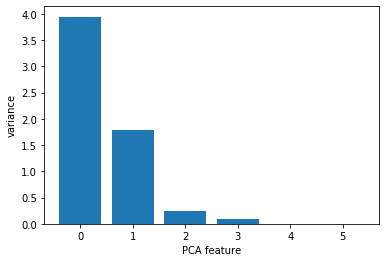import pandas as pd
import numpy as np
import matplotlib.pyplot as plt
import seaborn as sns


## Visualizing the PCA transformation

• Dimension reduction
• More efficient storage and computation
• Remove less-informative "noise" features, which cause problems for prediction tasks, e.g. classification, regression.
• Principal Component Analysis (PCA)
• Fundamental dimension reduction technique
• "Decorrelation"
• Reduce dimension
• PCA aligns data with axes
• Rotates data samples to be aligned with axes
• Shifts data samples so they have mean 0
• No information is lost
• PCA features
• Rows : samples
• Columns : PCA features
• Row gives PCA feature values of corresponding sample
• Pearson Correlation
• Measures linear correlation of features
• Value between -1 and 1
• Value of 0 means no linear correlation
• Principal components
• directions of variance
• PCA aligns principal components with the axes

### Correlated data in nature

You are given an array grains giving the width and length of samples of grain. You suspect that width and length will be correlated. To confirm this, make a scatter plot of width vs length and measure their Pearson correlation.

#### Preprocess

df = pd.read_csv('./dataset/seeds-width-vs-length.csv', header=None)

0 1
0 3.312 5.763
1 3.333 5.554
2 3.337 5.291
3 3.379 5.324
4 3.562 5.658
grains = df.values

from scipy.stats import pearsonr

# Assign the 0th column of grains: width
width = grains[:, 0]

# Assign the 1st column of grains: length
length = grains[:, 1]

# Scatter plot width vs length
plt.scatter(width, length)
plt.axis('equal');

# Calculate the Pearson correlation
correlation, pvalue = pearsonr(width, length)

# Display the correlation
print(correlation)

0.8604149377143466### Decorrelating the grain measurements with PCA

You observed in the previous exercise that the width and length measurements of the grain are correlated. Now, you'll use PCA to decorrelate these measurements, then plot the decorrelated points and measure their Pearson correlation.

from sklearn.decomposition import PCA

# Create a PCA instance: model
model = PCA()

# Apply the fit_transform method of model to grains: pca_features
pca_features = model.fit_transform(grains)

# Assign 0th column of pca_features: xs
xs = pca_features[:, 0]

# Assign 1st column of pca_features: ys
ys = pca_features[:, 1]

# Scatter plot xs vs ys
plt.scatter(xs, ys)
plt.axis('equal');

# Calculate the Pearson correlation of xs and ys
correlation, pvalue = pearsonr(xs, ys)

# Display the correlation
print(correlation)

4.85722573273506e-17## Intrinsic dimension

• Intrinsic dimension
• Intrinsic dimension = number of features needed to approximate the dataset
• Essential idea behind dimension reduction
• What is the most compact representation of the samples?
• Can be detected with PCA
• PCA identifies intrinsic dimension
• Scatter plots work only if samples have 2 or 3 features
• PCA identifies intrinsic dimension when samples have any number of features
• Intrinsic dimension = number of PCA features with signficant variance

### The first principal component

The first principal component of the data is the direction in which the data varies the most. In this exercise, your job is to use PCA to find the first principal component of the length and width measurements of the grain samples, and represent it as an arrow on the scatter plot.

plt.scatter(grains[:, 0], grains[:, 1])

# Create a PCA instance: model
model = PCA()

# Fit model to points
model.fit(grains)

# Get the mean of the grain samples: mean
mean = model.mean_

# Get the first principal component: first_pc
first_pc = model.components_[0, :]

# Plot first_pc as an arrow, starting at mean
plt.arrow(mean, mean, first_pc, first_pc, color='red', width=0.01)

# keep axes on same scale
plt.axis('equal');
plt.savefig('../images/pca-arrow.png')### Variance of the PCA features

The fish dataset is 6-dimensional. But what is its intrinsic dimension? Make a plot of the variances of the PCA features to find out. As before, samples is a 2D array, where each row represents a fish. You'll need to standardize the features first.

#### Preprocess

df = pd.read_csv('./dataset/fish.csv', header=None)

0 1 2 3 4 5 6
0 Bream 242.0 23.2 25.4 30.0 38.4 13.4
1 Bream 290.0 24.0 26.3 31.2 40.0 13.8
2 Bream 340.0 23.9 26.5 31.1 39.8 15.1
3 Bream 363.0 26.3 29.0 33.5 38.0 13.3
4 Bream 430.0 26.5 29.0 34.0 36.6 15.1
samples = df.loc[:, 1:].values

from sklearn.preprocessing import StandardScaler
from sklearn.pipeline import make_pipeline

# Create scaler: scaler
scaler = StandardScaler()

# Create a PCA instance: pca
pca = PCA()

# Create pipeline: pipeline
pipeline = make_pipeline(scaler, pca)

# Fit the pipeline to 'samples'
pipeline.fit(samples)

# Plot the explained variances
features = range(pca.n_components_)
plt.bar(features, pca.explained_variance_)
plt.xlabel('PCA feature')
plt.ylabel('variance')
plt.xticks(features);## Dimension reduction with PCA

• Dimension reduction
• Represent same data, using less features
• Important part of machine-learning pipelines
• Can be performed using PCA
• Dimension reduction with PCA
• PCA features are in decreasing order of variance
• Assumes the low variance features are "noise", and high variance features are informative
• Specify how many features to keep
• Intrinsic dimension is a good choice
• Word frequency arrays
• Rows represent documents, columns represent words
• Entries measure presence of each word in each document, measure using "tf-idf"

### Dimension reduction of the fish measurements

In a previous exercise, you saw that 2 was a reasonable choice for the "intrinsic dimension" of the fish measurements. Now use PCA for dimensionality reduction of the fish measurements, retaining only the 2 most important components.

#### Preprocess

scaler = StandardScaler()
scaled_samples = scaler.fit_transform(samples)

pca = PCA(n_components=2)

# Fit the PCA instance to the scaled samples
pca.fit(scaled_samples)

# Transform the scaled samples: pca_features
pca_features = pca.transform(scaled_samples)

# Print the shape of pca_features
print(pca_features.shape)

(85, 2)


### A tf-idf word-frequency array

In this exercise, you'll create a tf-idf word frequency array for a toy collection of documents. For this, use the TfidfVectorizer from sklearn. It transforms a list of documents into a word frequency array, which it outputs as a csr_matrix. It has fit() and transform() methods like other sklearn objects.

documents = ['cats say meow', 'dogs say woof', 'dogs chase cats']

from sklearn.feature_extraction.text import TfidfVectorizer

# Create a TfidfVectorizer: tfidf
tfidf = TfidfVectorizer()

# Apply fit_transform to document: csr_mat
csr_mat = tfidf.fit_transform(documents)

# Print result of toarray() method
print(csr_mat.toarray())

# Get the word: words
words = tfidf.get_feature_names()

# Print words
print(words)

[[0.51785612 0.         0.         0.68091856 0.51785612 0.        ]
[0.         0.         0.51785612 0.         0.51785612 0.68091856]
[0.51785612 0.68091856 0.51785612 0.         0.         0.        ]]
['cats', 'chase', 'dogs', 'meow', 'say', 'woof']


### Clustering Wikipedia part I

You saw in the video that TruncatedSVD is able to perform PCA on sparse arrays in csr_matrix format, such as word-frequency arrays. Combine your knowledge of TruncatedSVD and k-means to cluster some popular pages from Wikipedia. In this exercise, build the pipeline. In the next exercise, you'll apply it to the word-frequency array of some Wikipedia articles.

Create a Pipeline object consisting of a TruncatedSVD followed by KMeans. (This time, we've precomputed the word-frequency matrix for you, so there's no need for a TfidfVectorizer).

The Wikipedia dataset you will be working with was obtained from here.

from sklearn.decomposition import TruncatedSVD
from sklearn.cluster import KMeans
from sklearn.pipeline import make_pipeline

# Create a TruncatedSVD instance: svd
svd = TruncatedSVD(n_components=50)

# Create a KMeans instance: kmeans
kmeans = KMeans(n_clusters=6)

# Create a pipeline: pipeline
pipeline = make_pipeline(svd, kmeans)


### Clustering Wikipedia part II

It is now time to put your pipeline from the previous exercise to work! You are given an array articles of tf-idf word-frequencies of some popular Wikipedia articles, and a list titles of their titles. Use your pipeline to cluster the Wikipedia articles.

A solution to the previous exercise has been pre-loaded for you, so a Pipeline pipeline chaining TruncatedSVD with KMeans is available.

#### Preprocess

from scipy.sparse import csc_matrix

titles = documents.columns
articles = csc_matrix(documents.values).T

type(articles)

scipy.sparse.csr.csr_matrix
articles.T.shape

(13125, 60)
pipeline.fit(articles)

# Calculate the cluster labels: labels
labels = pipeline.predict(articles)

# Create a DataFrame aligning labels and titles: df
df = pd.DataFrame({'label': labels, 'article': titles})

# Display df sorted by cluster label
print(df.sort_values('label'))

    label                                        article
38      0                                         Neymar
36      0              2014 FIFA World Cup qualification
35      0                Colombia national football team
34      0                             Zlatan Ibrahimović
32      0                                   Arsenal F.C.
31      0                              Cristiano Ronaldo
30      0                  France national football team
39      0                                  Franck Ribéry
37      0                                       Football
18      1  2010 United Nations Climate Change Conference
17      1  Greenhouse gas emissions by the United States
16      1                                        350.org
15      1                                 Kyoto Protocol
19      1  2007 United Nations Climate Change Conference
13      1                               Connie Hedegaard
12      1                                   Nigel Lawson
11      1       Nationally Appropriate Mitigation Action
10      1                                 Global warming
47      1                                          Fever
14      1                                 Climate change
28      2                                  Anne Hathaway
27      2                                 Dakota Fanning
25      2                                  Russell Crowe
26      2                                     Mila Kunis
23      2                           Catherine Zeta-Jones
22      2                              Denzel Washington
21      2                             Michael Fassbender
20      2                                 Angelina Jolie
24      2                                   Jessica Biel
29      2                               Jennifer Aniston
51      3                                     Nate Ruess
52      3                                     The Wanted
53      3                                   Stevie Nicks
54      3                                 Arctic Monkeys
55      3                                  Black Sabbath
56      3                                       Skrillex
57      3                          Red Hot Chili Peppers
58      3                                         Sepsis
40      4                                    Tonsillitis
48      4                                     Gabapentin
46      4                                     Prednisone
45      4                                    Hepatitis C
49      4                                       Lymphoma
43      4                                       Leukemia
42      4                                    Doxycycline
41      4                                    Hepatitis B
44      4                                           Gout